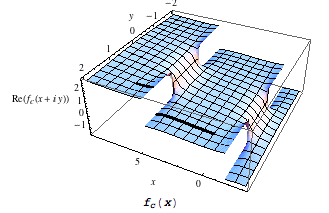There was a great blog post on the Wolfram Blog today on the Fundamental Theorem of Calculus and how they have to “tweak” the coding of definite integral evaluations to account for discontinuous anti-derivatives. The blog post also gives a few examples of calculus in the complex plane (that includes imaginary numbers in calculus) with a couple of cool graphics on calculus in the complex plane. Eventually, as part of a math or electrical engineering degree, students would take Complex Analysis, so I’m using this blog post as a way to give my calculus students a taste of what’s to come later on if they continue in mathematics.This is a great example of why it’s important for us (human beings) to continue to understand the underlying concepts of mathematics and not to just rely on computers to do everything for us. The majority of the blog post should be accessible for Calculus II students (or end-of-the-semester Calc I students), so send them along to this nice article and have them expand their horizons a bit!

Possibly Related Posts: